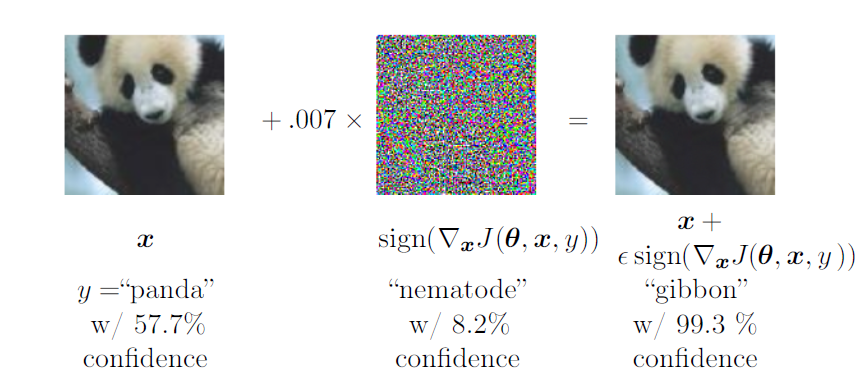# 深度学习：正则化

## 7.1 概念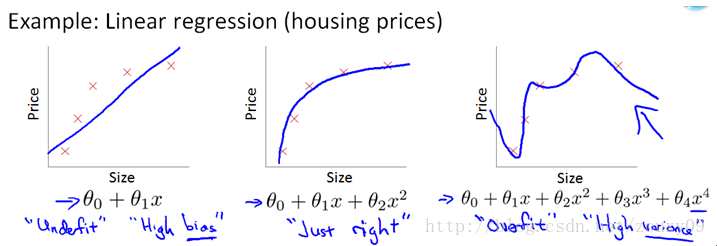• 欠拟合:实际的正例没有完全被包含在模型预测域。
• 绝佳：完全匹配了数据生成过程.
• 过拟合：模型的预测域包含了全部的正例，同时也包含了负例。

## 7.2 参数规范惩罚

• 向量$w$代表所有需要被规范惩罚的权重
• 向量 $\theta$代表所有参数，包括$w$和其他非正规化的参数

### 7.2.1 $L^2$ 参数正规化

L2范数是指向量各元素的平方和然后求平方根。我们让L2范数的规则项 $|W|_2$最小，可以使得W的每个元素都很小，都接近于0，但与L1范数不同，它不会让它等于0，而是接近于0。

#### 7.2.1.1 $L^2$如何实现参数调整

• 当 $\lambda _i\gg \alpha$ 时，正则化效果十分有限。
• 当 $\lambda _i\ll \alpha$ 时，将极大地改变 $w*$，几乎可以得到$w\sim 0，但是不为0$。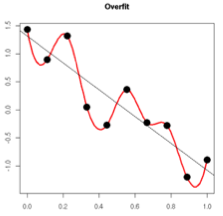### 7.2.2 $L^1$正则化处理

• $w_i\le\frac{\alpha}{H_{i,i}}$ 时， $w_i=0$ ,因为 $\widetilde J(w;X,y)$ 中的 $J(w;X,y)$ 在方向 $i$ 上完全被 $L^1$ 正则项覆盖。

• $w_i\gt\frac{\alpha}{H_{i,i}}$ 时，正则项没有将 $w_i$ 的最优置于0，而只是在该方向上移动了距离 $\frac{\alpha}{H_{i,i}}$

## 7.3 规范惩罚用作约束优化

• $\Omega (\theta)\gt k$时 $\alpha$ 增加
• $\Omega(\theta)<k$时 $\alpha$ 减小

## 7.4 正则化和受限问题

（多元）线性回归问题的损失函数为：

（可以看到，如果 $X^TX$ 是奇异的，将无法求解）

## 7.7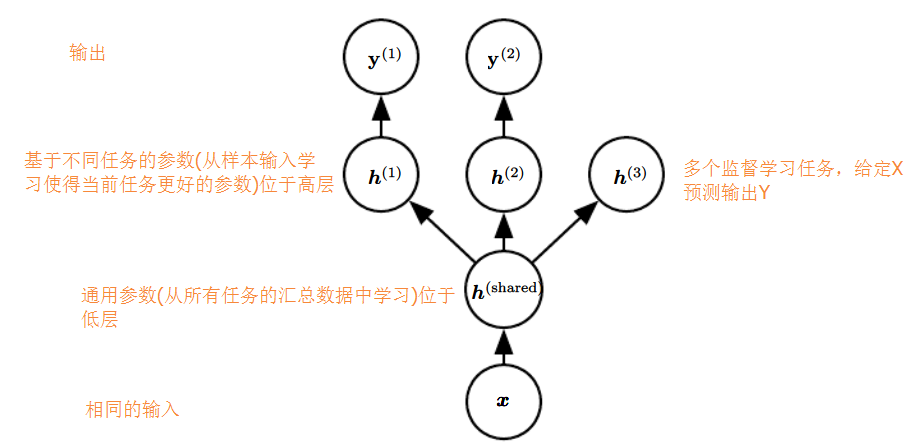## 7.8 提前终止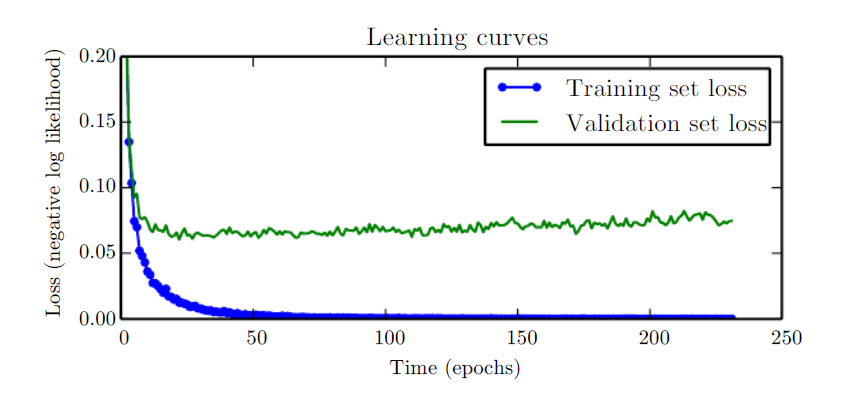• 在算法开始之前，先确定训练次数。
• 在训练过程中定期地运行验证，验证集可以比训练集数据量小。

• 几乎不改变算法过程，易于使用
• 提前终止可以很容易地与其他正则化方法结合使用。

• 需要不断保存最优模型参数，这是可以接收的，可以直接存放在磁盘上。
• 需要一个验证数据集，验证数据集不用于训练。

• 重新初始化模型，在所有数据集上重新训练，但只训练提前终止训练的次数。此时可以增加一些参数，因为数据更多（个人认为，其实是分两步走，第一步是用提前终止找到最优训练次数，第二次再完全训练）

• 保存第一次训练时的所有参数，并在所有数据上继续训练。此时无法知晓算法何时停止，但是可以观察验证数据集上的平均Loss，当其低于第一次训练的loss时停止。此方法可以避免第一次的重复计算，但是表现一般。

## 7.9 参数捆绑和参数共享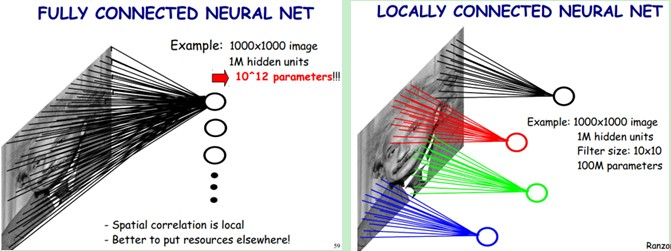## 7.10 稀疏表述

$L^1$ 正则化带来了稀疏项，是通过使得部分参数为0，而稀疏表述则直接让其中的表述元素直接为0.举例线性回归来说明这种差别:

## 7.11 集成学习方法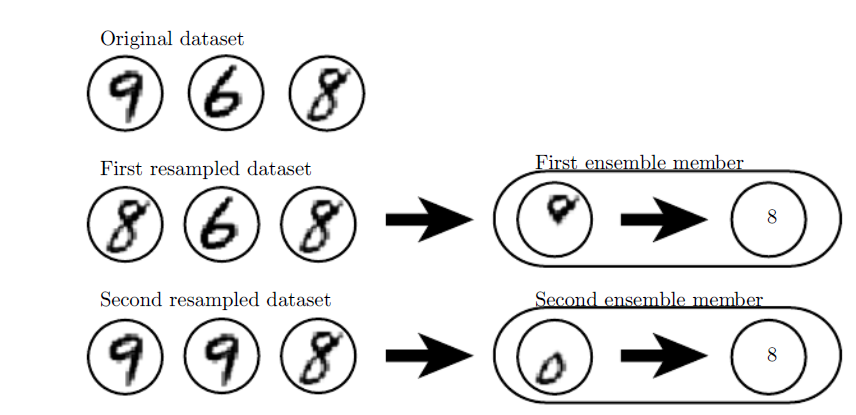## 7.12 dropout

dropout提供了一种计算量不大，但是强大的正则化方法。它是对一个模型族进行正则化处理。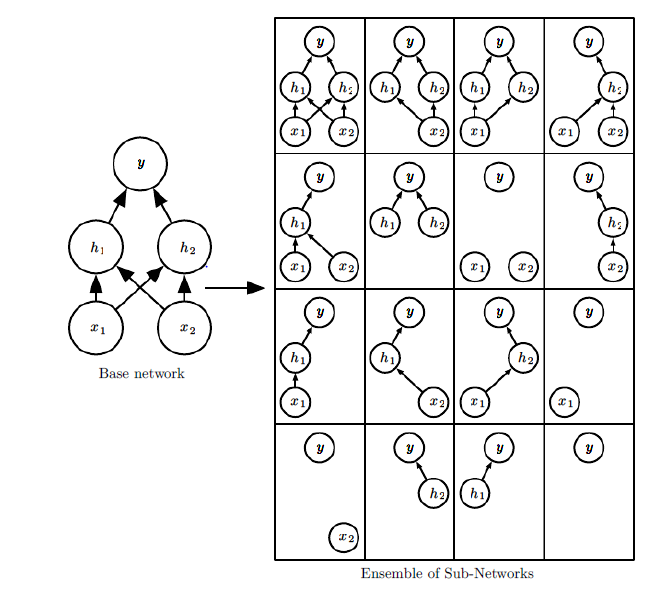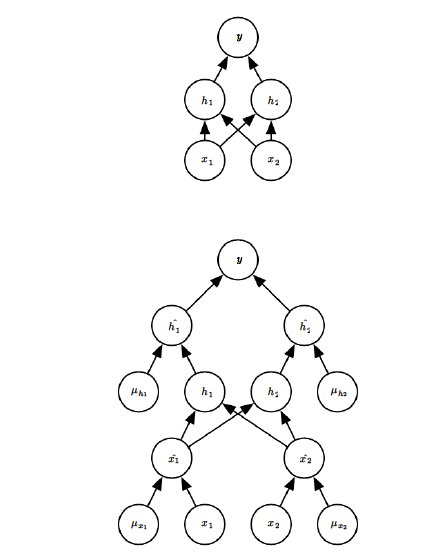$\frac{1}{k}\sum_{i=1}^kp^{(i)}(y\|x)$ dropout模型中每个由掩码向量 $\mu$ 定义的子模型定义了一个概率分布 $p(y|x,\mu)$，其inference是所有掩码概率分布的均值。所有掩码的算术均值如下:

dropout中可以通过估计一个模型的 $p(y|x)$ 来近似 $p_{ensemble}$ ，该模型包含了所有的神经元，但是每个神经元输出要乘以该神经元被保留的概率。此方法可以获得该神经元的期望输出。我们称此方法为scale inference rule，此方法只是一种经验上的技巧，并没有学术论证，但是实际效果很好。

## 7.13 对抗训练